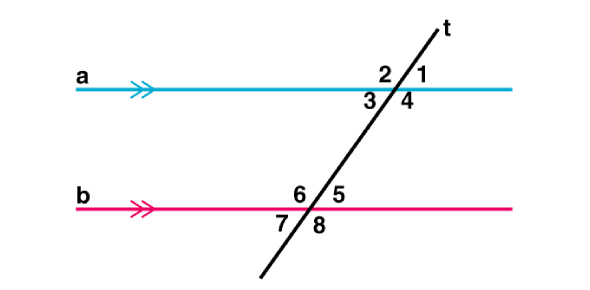# Lines And Angles For 9th-garde Students

6 Questions | Total Attempts: 433SettingsThis quiz is designed as a review of Lines and Angles found in Geometry, it is based on 9th-grade maths knowledge!

• 1.
What does this picture show?
• A.

Intersecting lines

• B.

Perpendicular lines

• C.

Parallel lines

• 2.
What is this picture showing?
• A.

Parallel lines

• B.

Perpendicular lines

• C.

Intersecting lines

• 3.
What type of angle is this?
• A.

Right angle

• B.

Acute angle

• C.

Obtuse angle

• 4.
What type of angle is this?
• A.

Acute angle

• B.

Right angle

• C.

Obtuse angle

• 5.
When it is 10:30, what kind of angle is formed by the hands of the clock?
• A.

Acute

• B.

Obtuse

• C.

Right

• 6.
Which figures below show pairs of lines that appear to be parallel?
• A.

Figure 1

• B.

Figure 2

• C.

Figure 3Back to top